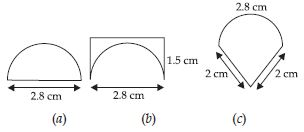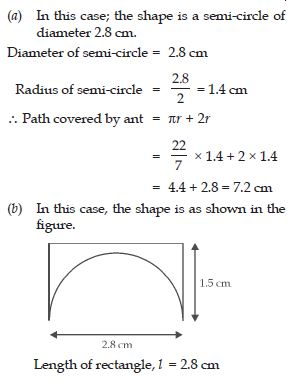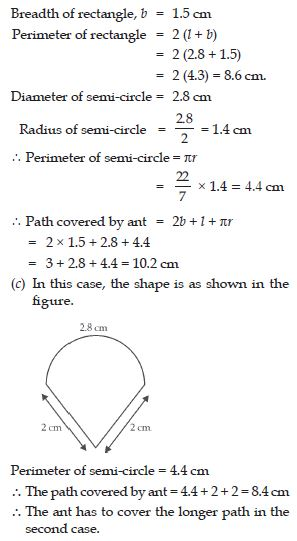# NCERT Solutions for Class 8 Math Chapter 11 - Mensuration

##### Question 1:

A square and a rectangular field with measurements as given in the figure have the same perimeter. Which field has a larger area?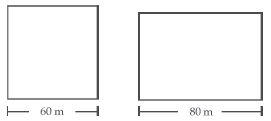Side of square = 60 m
Perimeter of square = 4 × side
= 4 × 60 = 240 m
Length of rectangle = 80 m
Perimeter of rectangle = Perimeter of square
2 (a + b) = 240
2 (80 + b) = 240
160 + 2b = 240
2b = 240 – 160 = 80
$⇒$ b = 40
$\therefore$ Breadth of rectangle = 40 m
Now, area of square = (side)2
= (60)2 = 3600 m2
Area of rectangle = a × b
= 80 × 40 = 3200 m2
$\therefore$ Square has the larger area.

##### Question 2:

Mrs. Kaushik has a square plot with the measurement as shown in the figure. She wants to construct a house in the middle of the plot. A garden is developed around the house. Find the total cost of developing a garden around the house, at the rate of ₹ 55 per m2.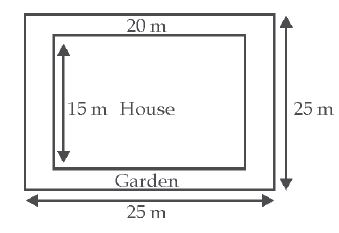Side of square plot = 25 m
Area of square plot = (side)2
= (25)2 = 25 × 25 = 625 m2
Length of house = 20 m
Breadth of house = 15 m
Area of house = 20 × 15 = 300 m2
$\therefore$ Area of Garden = Area of Plot – Area of house
= 625 – 300 = 325 m2
Cost of developing the garden at the rate of
₹ 55 per m2
= 325 × 55 = ₹17875.

##### Question 3:

The shape of a garden is rectangular in the middle and semi-circular at the ends as shown in the diagram. Find the area and the perimeter of this garden. [Length of rectangle = 20 – (3.5 + 3.5) = 13 metres].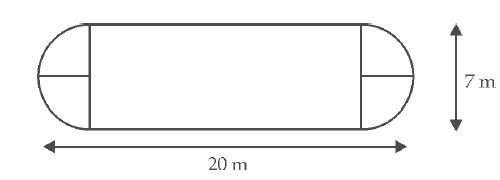##### Question 4:

A flooring tile has the shape of a parallelogram whose base is 24 cm and the corresponding height is 10 cm. How many such tiles are required to cover a floor of area 1080 m2?##### Question 5:

An ant is moving around a few food pieces of different shapes scattered on the floor. For which food-piece would the ant have to take a longer round? Remember, circumference of a circle can be obtained by using the expression c = 2πr; where r is the radius of the circle.